Courses

# Important Formula: Averages CAT Notes | EduRev

## Quant : Important Formula: Averages CAT Notes | EduRev

The document Important Formula: Averages CAT Notes | EduRev is a part of Quant category.
All you need of Quant at this link: Quant

• Simple Average =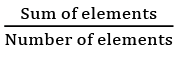• Weighted Average =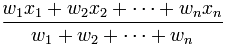• Arithmetic Mean = (a1 + a2 + a3 ….an) / n
• Geometric Mean =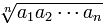• Harmonic Mean =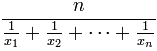For two numbers a and b

⇒ AM = (a + b)/2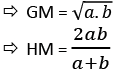EduRev's Tip:

• AM ≥ GM ≥ HM is always true. They will be equal if all elements are equal to each other. If I have just two values then GM2 = AM x HM
• The sum of deviation (D) of each element with respect to the average is 0

⇒ D = (x1 - xavg) + (x2 - xavg) + (x3 - xavg) ... + (x1 - xavg) = 0

• 𝑥avg =  (xassumed avg + (Deviation/No. of elements))

Median of a finite list of numbers can be found by arranging all the observations from lowest value to highest value and picking the middle one.
Mode is the value that occurs most often

Offer running on EduRev: Apply code STAYHOME200 to get INR 200 off on our premium plan EduRev Infinity!

,

,

,

,

,

,

,

,

,

,

,

,

,

,

,

,

,

,

,

,

,

;